Question

# you weigh out 7.25 grams of NaCl and dissolve this sample in 50 mL of deionized...

you weigh out 7.25 grams of NaCl and dissolve this sample in 50 mL of deionized water, and then you put it in an empty volumetric flask weighing 57.221 g. After the whole thing has dissolved, you add water all the way up to the 100.0 mL mark of the volumetric flask (flask and solution together weighs 162.9145 g.) what’s the mass % of NaCl in this solution?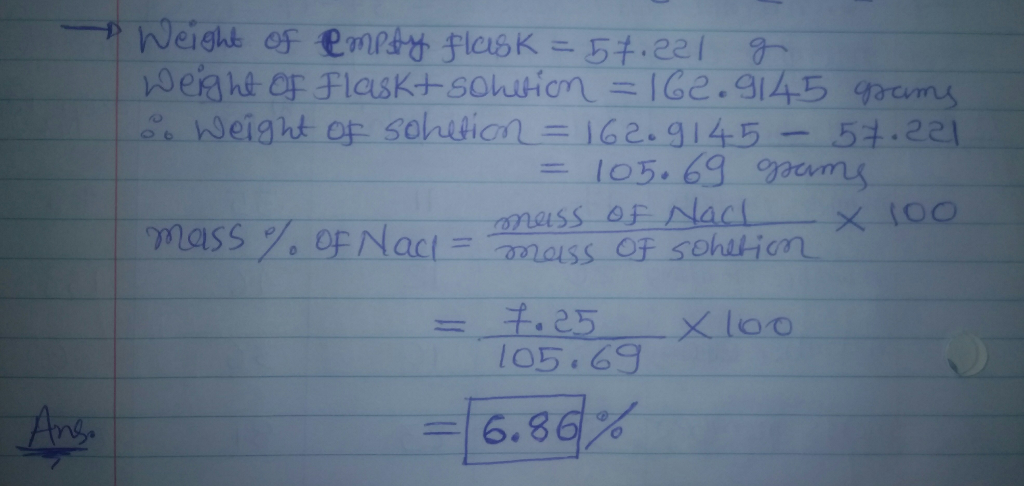#### Earn Coins

Coins can be redeemed for fabulous gifts.

Similar Homework Help Questions
• ### A student weighs out 14.6 g of AlBrz, transfers it to a 300 mL volumetric flask,...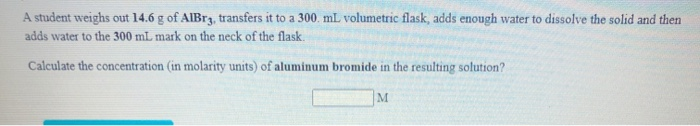A student weighs out 14.6 g of AlBrz, transfers it to a 300 mL volumetric flask, adds enough water to dissolve the solid and then adds water to the 300 mL mark on the neck of the flask. Calculate the concentration (in molarity units) of aluminum bromide in the resulting solution? Calculate the mass, in grams of sodium iodide that must be added to a 250 mL volumetric flask in order to prepare 250 mL of a 0.138 M aqueous...

• ### Can you please tell me the grams i need for these 2 solutions? Thank You Weigh...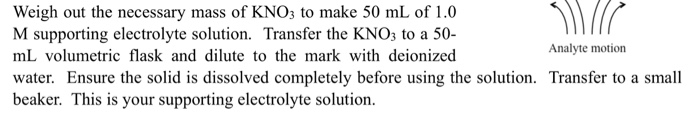Can you please tell me the grams i need for these 2 solutions? Thank You Weigh out the necessary mass of KNO3 to make 50 mL of 1.0 M supporting electrolyte solution. Transfer the KNO3 to a 50- mL volumetric flask and dilute to the mark with deionized Analyte motion water. Ensure the solid is dissolved completely before using the solution. Transfer to a small beaker. This is your supporting electrolyte solution. Weigh out the necessary mass of K3Fe(CN)6 to...

• ### 6.52 grams of sodium hydrogen carbonate is weighed into a 150 ml beaker and dissolved in 100 ml of deionized water. The...

6.52 grams of sodium hydrogen carbonate is weighed into a 150 ml beaker and dissolved in 100 ml of deionized water. The solution is then quantitatively transfer to a 250.0 mL volumetric flask and filled to the line with deionized water. What is the molarity of hydrogen carbonate in the volumetric flask?

• ### In the laboratory, a student adds 19.3 g of potassium acetate to a 125 mL volumetric...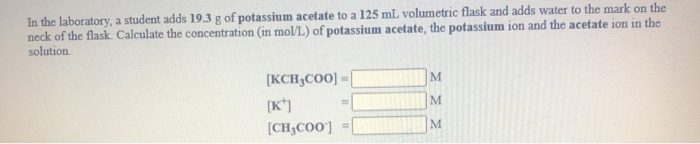In the laboratory, a student adds 19.3 g of potassium acetate to a 125 mL volumetric flask and adds water to the mark on the neck of the flask. Calculate the concentration in mol/L) of potassium acetate, the potassium ion and the acetate ion in the solution [KCH3COO) - [K] [CH3C00] = 1 A student wishes to prepare 300-ml of a 0.236 M iron(III) acetate solution using solid iron(III) acetate, a 300-ml volumetric flask, and deionized water (a) How many...

• ### Please do 2,3 and 4 Experiment 3 Pr 1. A student weigh be 98.595 oth mark,...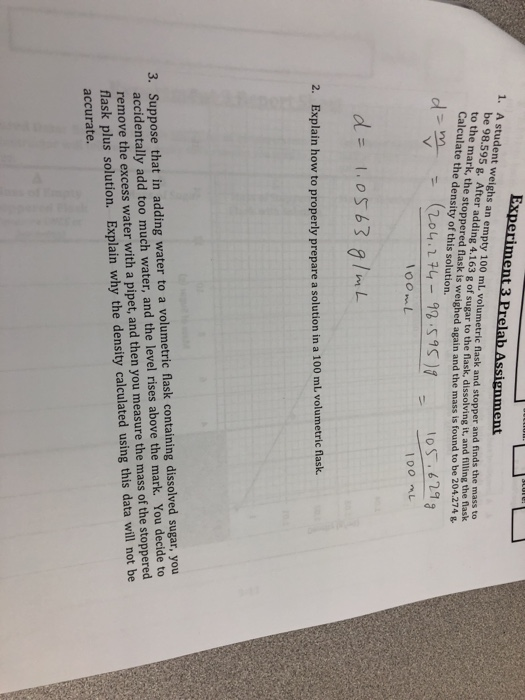Please do 2,3 and 4 Experiment 3 Pr 1. A student weigh be 98.595 oth mark, the stoppered flask is weighed again and the mass is found to be 204.274 & ment s an empty 100 mL. volumetric flask and stopper and finds the mass to g After adding 4.163 g of sugar to the flask, dissolving it, and filling the flask Calculate the density of this solution dm oS 629 2. Explain how to properly prepare a solution in...

• ### A student prepares 100.00 mL of 0.133 M NaCl in water. 12.5 mL of the solution...

A student prepares 100.00 mL of 0.133 M NaCl in water. 12.5 mL of the solution is then measured with a 25 mL graduated cylinder and transferred to a 50.00 mL volumetric flask which is then filled to the mark with deionized water. what is the molarity of NaCl in the 50.00 mL volumetric flask?

• ### How much NaCl would you dissolve and dilute to the 100.00 mL mark in a volumetric...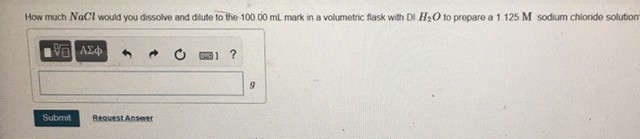How much NaCl would you dissolve and dilute to the 100.00 mL mark in a volumetric flask with DI H2O to prepare a 1.125 M sodium chloride solution IV AED O ? Submit Besuest Answer

• ### A student weighs out a 3.07 g sample of NiF2, transfers it to a 250. mL...

A student weighs out a 3.07 g sample of NiF2, transfers it to a 250. mL volumetric flask, adds enough water to dissolve it and then adds water to the 250. mL tick mark. What is the molarity of nickel(II) fluoride in the resulting solution?

• ### A student weighs out a 14.4 g sample of KBr, transfers it to a 500. mL...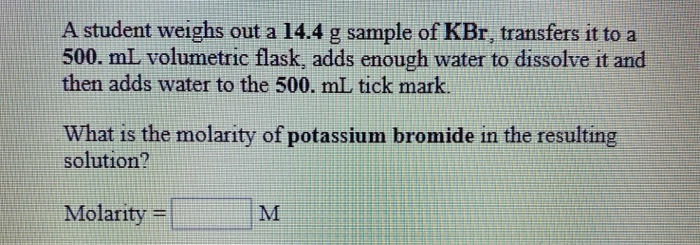A student weighs out a 14.4 g sample of KBr, transfers it to a 500. mL volumetric flask, adds enough water to dissolve it and then adds water to the 500. mL tick mark. What is the molarity of potassium bromide in the resulting solution? Molarity = M

• ### A student wishes to prepare 300-mL of a 0.236 M iron(III) acetate solution using solid iron(III)...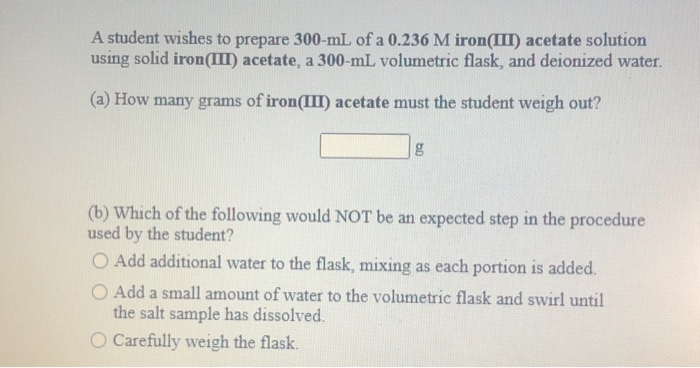A student wishes to prepare 300-mL of a 0.236 M iron(III) acetate solution using solid iron(III) acetate, a 300-ml volumetric flask, and deionized water. (a) How many grams of iron(III) acetate must the student weigh out? (b) Which of the following would NOT be an expected step in the procedure used by the student? O Add additional water to the flask, mixing as each portion is added. O Add a small amount of water to the volumetric flask and swirl...